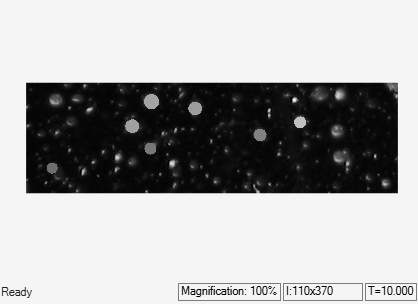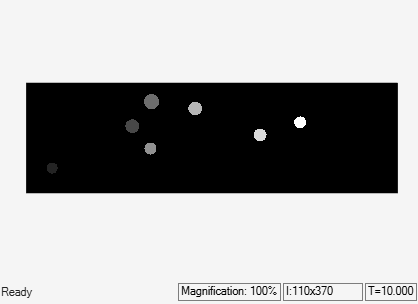Replace Intensity Values in ROI with its Maximum Value

This example shows how to find maximum intensity value of region of interests (ROI) in the input image and replace the pixels in the ROI with its maximum value

The input to the model is the original image, label matrix, and the label values. The label matrix contain the desired ROIs in the input image. Load the label matrix into MATLAB workspace.

Find the unique label values in the label matrix. The label value 0 corresponds to the background and must be ignored.

lb = unique(Labelmatrix);
label = lb(2:end);

Example Model

Open the Simulink model. The model reads the input image using the Image From File block.

open_system('ex_blk2dmaximum.slx');The model computes the maximum intensity value for each ROI and replaces all the pixel values in the ROI to maximum value. The model computes the maximum value for each ROI individually by setting these 2-D Maximum block parameters to the specified value,

• Set the Mode parameter to Value.

• Set the Find the maximum value over parameter to Entire input.

• Set the Enable ROI processing parameter and set the ROI type parameter as Label matrix.

The Matlab Function block replaces the individual ROI's with its maximum intensity value and outputs the resultant image.

Simulate and Display Results

Run the model and display the images using Video Viewer block.

sim('ex_blk2dmaximum.slx');